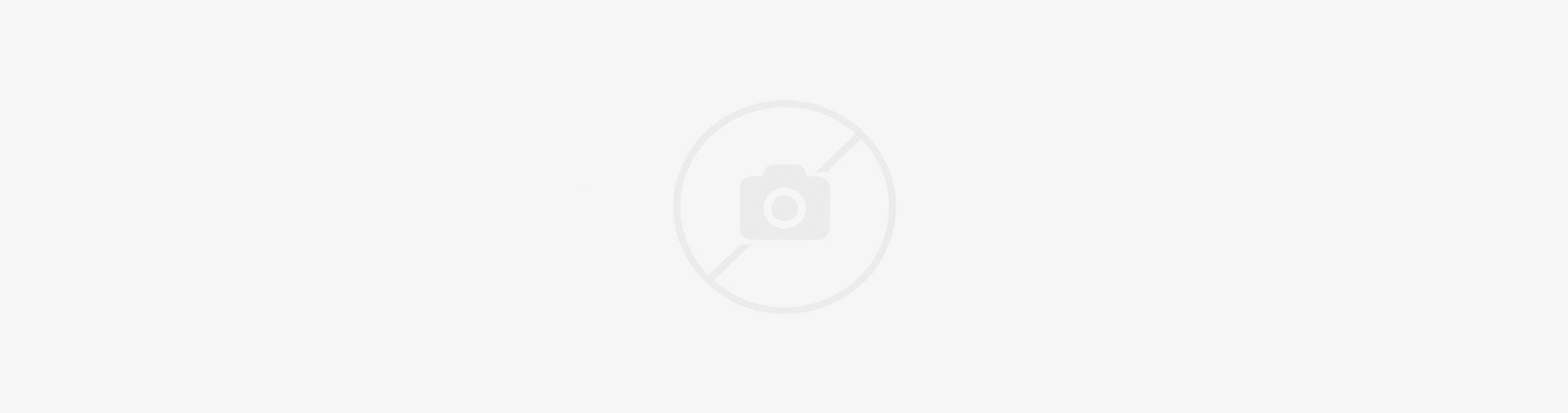### Code:

``` [product title="Featured collection" limit="4" cols="3" cols_mb="4" cols_xs="6" pagination="view-all" layout="layout1" handle_collection="einzel-sticker" style="" vendors="false" countdown="false" countdown_style="dark" equal="true" size="nt_contain" ratio="ratio1_1" position="8" ] ```

### Code:

``` [product title="Featured collection owl" limit="8" cols="4" autoplay="false" autoplaySpeed="6000" infinite="false" arrows="true" dots="false" 1024="3" 600="2" 480="2" pagination="" layout="layout2" handle_collection="" style="" vendors="false" countdown="false" countdown_style="dark" equal="false" size="nt_cover" ratio="ratio_nt" position="8" ] ```

### Code:

``` [lookbook title="Lookbook" total="0" ] ```

### Demo:

#### Lookbook